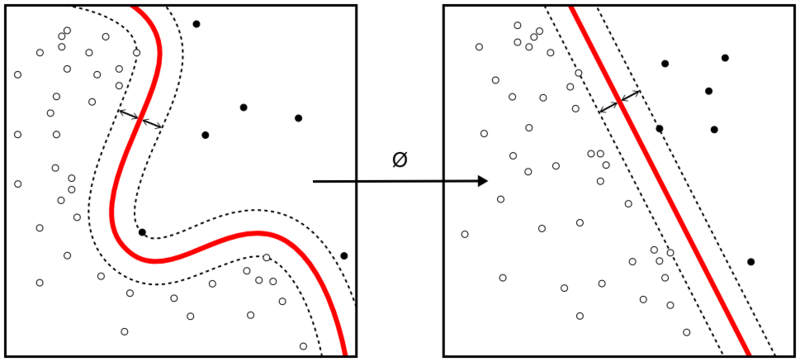## How to program the Support Vector Machines algorithm

Support Vector Machine is one of the most commonly used supervised machine learning algorithms for data classification. A binary classifier, the support vector machine algorithm works in vector space to sort data points by finding the best hyperplane separating them into two groups. Thanks to its reliance upon vectors, it finds frontiers between groups of data points even in nonlinear patterns and features spaces of high dimensions.Continue reading “How to program the Support Vector Machines algorithm”

## How to program the K Nearest Neighbors algorithm

K Nearest Neighbors is a popular classification algorithm for supervised machine learning. It permits to divide data points into groups, defining a model that will then be able to classify an unknown data point in one group or another. The K parameter, defined during programming, allows the algorithm to classify unknown data points by examining the K closest known data points.Continue reading “How to program the K Nearest Neighbors algorithm”

## Programming a simple classifier with TensorFlow

TensorFlow is an open-source machine learning framework developed by Google. It relies upon Tensors (multi-dimensional arrays) which empower a wide range of API to develop machine learning applications, primarily deep neural networks. TensorFlow is commonly used in machine learning practice, so better start using it already.

Thankfully the TensorFlow website provides a guide for programmers as well as detailed tutorials. Here is the basic tutorial to get get started with TensorFlow. To accompany programmers, Google cloud has also created a series of videos on machine learning and TensorFlow.

This next video is going over the basic tutorial with iris flowers images classification. Yufeng Guo walks us through the initial tutorial to develop a linear model to classify flowers, corresponding to the explanations and code available in the page “getting started with TensorFlow: Premade Estimators” and aimed at readers who have some experience in machine learning.

Note: to get this tutorial running well, you will need to have a Python IDE (such as PyCharm, or a Jupyter notebook) with a virtual environment loaded with the TensorFlow, Pandas and Numpy librairies. You will also need a Git client software (Git for Windows if you’re using Windows) to download the data from GitHub. You may use Anaconda to properly load the librairies in your Python IDE.

## 17. Learning: Boosting

“Wisdom of a weighted crowd of experts”

## Classifiers

Classifiers are tests that produce binary choices about samples. They are considered strong classifiers if their error rate is close to 0,  weak classifiers if their error rate is close to 0.5.

By using multiple classifiers with different weights, data samples can be sorted or grouped according to different characteristics.

## Decision tree stumps

Aside from classifiers, a decision tree can be used to sort positive and negative samples in a 2-dimension space. By adding weights to different tests, some samples can be emphasized over the others. The total sum of weights must always be constrained to 1 to ensure a proper distribution of samples.

## Dividing the space

By minimizing the error rate of the tests from the weights, the algorithm can cut the space to sort positive and negative examples.

## No over fitting

Boosting algorithms seems not to be over fitting, as the decision tree stumps tends to be very tightly close to outlying samples, only excluding them from the space.